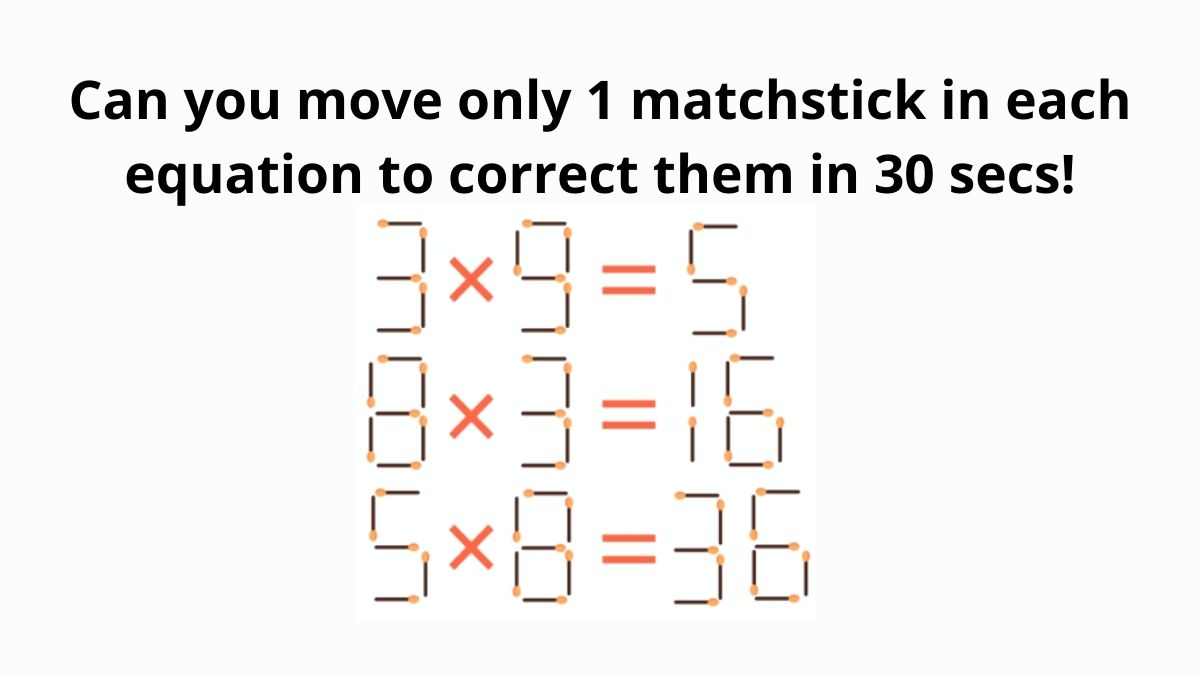# Brain Teaser Puzzle: Can you move only 1 Matchstick in each equation to correct them in 30 secs?

Brain Teaser Puzzle: In this Quiz, try to move only 1 matchstick in each equation to correct them given in the image. Can you solve this puzzle in 30 Seconds?Can you move only 1 Matchstick in each equation to correct them in 30 secs?

Can you move only 1 Matchstick in each equation to correct them in 30 secs? This brain teaser is for those who enjoy solving analytical puzzles and games. Brain teasers are mainly a trickier version of riddles and puzzles, as these brain games are solved with lateral thinking. While solving these, you need to analyze a little differently and out of the box. For coming on to the solution, you need to use a creative mind as the answer won’t be right in front of you. So, we have come up with an interesting brain-teaser where you need to move only 1 matchstick in each equation to correct them in the image.

Can you spot an old woman or a young girl hidden in this Century Old Optical Illusion?

## Move only 1 matchstick in each equation to correct them in 30 Seconds

Test your IQ by Spotting all 7 People & a Cat in this Optical Illusion

In the above image, you need to move only 1 matchstick in all of the three equations such that it fixes and corrects the equations. An alert mind can solve this puzzle within 20 seconds. You need to analyze the Matchsticks structure carefully shown in the image. As a heads up, the answers to this brain teaser have been given right below the question, so make sure you don’t scroll too far and cheat!

Faces you see first in this optical illusion tell a lot about your personality

Hint: Apply the rule: LHS = RHS.

Check A 900-Year-Old Optical Illusion revealing your Personality Traits

In this brain puzzle, you need to move only 1 matchstick in all of the three equations such that it corrects the equations. We can see that initially, all three equations are incorrect.

First face you see in this optical illusion reveals what you crave in a Romantic Relationship

If we look at the 1st equation carefully, we can see that if we move one match from the 9 so that it turns out "3 x 3", and put it to the 5 on the right so that it becomes a 9, we get the correct equation: 3 x 3 = 9.

Optical Illusion: Face you see first reveals what you Hate most about your Partner's Personality

In the second equation, the match is shifted in number 3 such that it becomes 2. The correct equation will be: 8 x 2 = 16.

Test your IQ by Spotting Hidden Panda in this Zig-Zag Optical Illusion

Well, in the third equation, removing the match from the number 8 and attaching it to the five below, we get another fair equality: 6 x 6 = 36.

Test your IQ by spotting all B’s in the group of D’s in this Optical Illusion

Stairs Optical Illusion: Can you guess in 10 seconds which way is the object moving?

Another answer to this matchstick brain teaser can be:

In the second equation, the match should be taken from the eight and added to the number 6. The correct equation will be 6 x 3 = 18.

Using lateral thinking will help you to derive answers in such brain teasers. This riddle was tricky but simple as it needs less time and brain power to solve. But it sure feels great when you figure out the answer in a few seconds.

Get the latest General Knowledge and Current Affairs from all over India and world for all competitive exams.
खेलें हर किस्म के रोमांच से भरपूर गेम्स सिर्फ़ जागरण प्ले पर